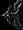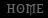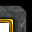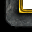# General - Balancing

Tutorial By Awestruck

When I say balancing, I don’t mean making two units with the same gold/lumber cost equally powerful. That’s simple. In fact; here’s the program that’ll do that for you. What I want, is to know how much more a unit will be worth if I change one of its aspects (like armor, damage or whatever), or even what a unit made from scratch should cost - in any map.

The ideas to do this originated when I myself needed to balance a map of mine. I tried to come up with the simplest way to figure out how to ‘rate’ different values of a unit.

Why? Because if I did, I could save a lot of time on all future balancing.

Chapter 1: Theory
Chapter 2: Magic Numbers
Chapter 3: How to use the Numbers
Chapter 4: Exceptions and Limitations
Chapter 5: How to find the Magic Numbers
Chapter 6: How to find other Magic Numbers
Chapter 7: Using Magic Numbers in Non-Melee Maps
Chapter 8: Using Magic Numbers in TDs
Conclusion

Chapter 1: Theory

The theory behind this entire tutorial is that for any unit (even buildings), each aspect (being damage, health, speed, abilities, and so on) represent a portion of that unit’s gold and lumber cost. If you know how big this portion is, you can find out how the two numbers behave to each other (how to find one via the other).

In other words, we want to find a constant that, when applied to the damage output, or the hp of a unit, the value, in gold, of that trait would be returned. (Mathematicians will know that this can’t be done unless f(x) = kx, x = value of aspect, f(x) = gold cost of aspect, but the values I’ve found seem to follow this form quite accurately, more on that in chapter 5)

The beauty of this is that even though you’ve understood nothing I’ve said so far, you could still use my discoveries to help balance your custom maps.

Chapter 2: Magic Numbers

I have found the factors for two unit-traits. I’ll call them ‘Magic Numbers’ for convenience (or just for fun).
Here they are:

Value of 1 dps: 5,3544563483342
Value of 1 effective hit point: 0,18159375101708

These numbers are the values of respectively 1 damage per second, and 1 effective hit point as blizzard seem to have meant them for units. They can be used to find the gold cost you should use for a unit (not building) in a melee map. Of course there are similar values for other aspects; but these two seemed to me to be the most important.

Chapter 3: How to use the Numbers

Now I’ll explain how to use these numbers to get an amount of gold returned. Depending on your experience with math and with the world editor, you won’t need to read much/any of this.

But; if you wanted to figure out how to price a Footman for instance, this is what you would do:

First you need to find the dps of the footman. This is the equation to get the dps of any unit:
(2*BaseDmg+NumberOfDice+SidesPerDie)/(CooldownTime*2)
For a Footman:
(2*11+1+2)/(2*1.35) = 25/(2*1.35) = 9.26 (rounded up here, you will get infinate decimals on a calculator)

And the equation for effective hp:
HitPoints*(Armor*0.06+1) note: the value ‘0.06’ is the ‘armor-multiplier’.
For a Footman:
420*(2*0.06+1) = 420*1.12 = 470.4 (this time only one decimal)

Believe it or not, that’s the hard part. The ‘value’ of the dps for any unit is:
Dps*MagicDpsNumber (This number is the one I gave you in chapter 2)
For a Footman:
9.26*5.35 = 49.58 (the value, in gold, of the damage a footman does)

The ‘value’ of the effective hp for a unit is:
Hp*MagicHpNumber (The other number from chapter 2)
For a Footman:
470.4*0.18 = 85.42 (the value, in gold, of the health of a footman)

Notice: the amount of decimals used depend on what I think is prettier. When doing the actual calculations, you should use as many decimals as possible.

So! The cost of this ‘Footman-Unit’ should be 85.42 + 49.58 = 135.00 gold. Since we cheated and used a unit that already has a price from Blizzard, we can check if Blizzard agrees. And I’ll give a cookie to anyone who can show me a non-photoshopped default value of the Footman’s gold cost that isn’t 135. (You can go and check in the World Editor right now ;))

Chapter 4: Exceptions and Limitations

Alright, I know this may seem all religious and god-sent, with the ‘Magic Numbers’ and exact results. But truthfully, the footman-result isn’t very impressive because of the way I found the numbers. Usually the found gold cost of a unit (if tested on other blizz units) is off by a couple gold. You can see why when I take the divinity out of those ‘Magic Numbers’ and explain how to find them in chapter 5.

Listed here are some very important things to keep in mind when calculating the value of a custom unit using the Magic Numbers. First; they assume that the properties of a unit are limited to:

Damage – Damage Base, Number of Dice, Sides per Die
(Other factors that effect damage, like abilities or items, can be included here easily)

Effective hit Points – Hit Points Maximum (base), Defence Base
(Again, factors like abilities and items can be included in calculating this value)

The numbers do not take into consideration:

Range
Abilities (that are more abstract than just changing dps and hp)
Movement Speed (that is different from 270, or whatever speed you used to find your ‘Magic Numbers’ in case you didn’t use mine)
Flying/Ground movement
Upgrades (although this shouldn’t have a very large impact as long as the upgrades are properly balanced)

For these things you need to figure out for yourself what they are worth. Either just add a few gold to the price according to what seems fair, or try to guess/decide what the cost of the aspect should be. It is usually a factor you need to multiply on either the base value you found of the unit (like when we found the base value of the Footman), or, in some cases, just the value of the dps or effective hp (there is an example of this in the TD section).

Also, I’ve not mentioned the ‘lumber phenomenon’ yet. I haven’t given this much thought, but it seems that lumber has just about the same value as gold, and is only in the game for flavour. What this means is that if you get the value of a unit in your map to be 500, you could set it to be 300 gold and 200 lumber, or whatever combination suits you. Don’t take my word for it though.

Chapter 5: How to find the Magic Numbers

To follow me in this section, you should have at least some basic knowledge of math (probably more).

So, as you can see the two ‘Magic Numbers’ are constants. To find them, we put ourselves in the position I was in before I found them. So we give them names instead of values.

D = value of one dps
H = value of one effective hit point

Those are our goals. We’ll find them through other known values. To do this we have to make two assumptions.

-The numbers are indeed constants. This is only the case if (as mentioned earlier) f(x) = kx
-The constants are true for all units.

Are these assumptions fair? No. Can we prove them later? No. But they do seem to be correct for these unit-aspects (dps and effective hit points) and that satisfies me. Feel free to test them on any unit.

I found them by solving two equations with two unknowns. To do this we need to set up two equations. Here are the ones I used:

D*9.26 + H*470.4 = 135
And
D*12.19 + H*742 = 200

The first equation is the same one we used to find the gold cost of the footman, except what values are unknown are different now. The second equation is the same as the first, except it uses the values for a grunt. I used these units because they are very simple. They are melee attackers with no special abilities.

Moving on we need to isolate D or H in one of the two equations. I’m actually horrible at math, especially at solving equations, so I just got my calculator to solve this for me, getting:

D = 14.58 – 50.80*H

That is simply D isolated in the first equation (the one for the footman).
You then put that expression of your D-value into the second equation:

(14.58 – 50.80*H)*12.19 + H*742 = 200 =>

H = 0.1815

Yay! One down :D
Second one is easier now that we have this value. We simply put our newly found value for H into the one of the two equations. Either way you’ll get the same result. Here I used the Footman-equation:

D*9.26 + 0.1815*470.4 = 135 =>

D*9.26 = 135 - 0.1815*470.4 =>

D = (135 - 0.1815*470.4)/9.26 = 5.354

Yay! There we go, both values found. I’ve proved that these are the values for the footman and the grunt, but not for other units. I don’t claim that they are correct for other units, but that they are very close.

Chapter 6: How to find other Magic Numbers

First, note that the 'Magic Numbers' are actually a constant value in a function. In chapter 2 I could have written the numbers in the form:

f(x) = kx , where k is a constant ,x is the unit-value and f(x) is the gold cost of x

I chose not to do this to avoid confusion among people not very good with math. This however may confuse people into thinking that the “relations” are numbers, instead of functions. They are not, and for different values are potentially different functions. Also, I think one function could refer to another. I'll demonstrate how later in this chapter.

To find the values of other unit-aspects you have to make sure to compensate for every one of them for the unit you use. For instance if you wanted to find what it’s worth to be able to fly, you could use a gargoyle and the equation: (value of gargoyle’s dps+hp)+(value of flying)=(bilzz’s value of gargoyle)?

Nope, you can’t. Because the speed of the Gargoyle is 350, which is not the base value of 270 (that we assumed was the base when we used the footman and grunt to find the two constants that are also used in this equation) and has to be compensated for, and it even has a ranged attack. So that’s an equation with three unknowns, not one.

And the form of this equation probably can’t be as simply set up as the two for dps and hp.

As I indicated in the beginning of the chapter, f(x) = UnitGoldCost can contain internal functions. Here is a guess as to how the gargoyle equation could look. Note that x represents different variables here.

D(d) = price of dps, d = dps
H(h) = price of effective hp, h = effective hp
S(x) = price of speed - BaseSpeed
R(x) = price of additional range
K1 = the flying-constant, probably between 1 and 2

f(x) = K1*S(R(D(d))+H(h))

More functions can then be added to f(x) to complete an equation that will return the total gold cost of any unit. To do this you would have to find a model for each function including f(x) = UnitGoldCost which will be quite chalanging.

Chapter 7: Using Magic Numbers in Non-Melee Maps

So, the numbers are constant. Meaning that you can change them, but then you have to change all the aspects of all your units. So if you wanted your units to cost huge amounts of gold, you could multiply each of the numbers by 10 for instance. I tend to like things costing less in my maps, so I will probably divide the constants with about 4.

You shouldn’t create units and use them to find ‘Magic Numbers’. The numbers are what link the stats of all units, and the whole idea behind finding them is that Blizzard knew what they were doing when they finished balancing Warcraft III.

Chapter 8: Using Magic Numbers in TDs

Towers in TDs are quite easy to balance. What makes them easy is that the attackers only have one aspect to balance – effective hp, and you don’t need to think about this for defenders. This obviously isn’t true if attackers are able to attack towers. Also, the amount of variables for each encounter are very few (it’s a very predictable game type in terms of combat).

For towers you basically just need to set the price of the dps. After this you can think about the range. Don’t think of it (the range) as a value, but as a factor that helps determine for how long the tower can attack a runner. If for instance you assume that the length of the line the runner will walk while in range of the tower is the diameter (range*2) of the circle within reach of the tower, you can calculate this time.

t = d/v

t is time, d is distance, and v is velocity (or speed, measured in d/t. In our case, the speed of the runner).

So if a tower has a range of 700 (measured in war3 in pixels) when a unit walks through it in a straight line with a speed of 300 (pixels/second), the unit would be within range of the tower for:

t = 2*700/300 = 2 + (1/3) = 4.667 seconds

Using the attack speed of the tower you can calculate the exact amount of times the tower will be able to attack this unit. This value is:

A = t/s

A is amount of attacks, t is time, and s is attack speed.

So for the previous value of 4.667 seconds, if the tower’s attack speed was 1.5 the amount of attacks would be:

4.667/1.5 = 3.111

Of course for one runner the tower would only attack one time, but if 20 units were to run through, it would attack ‘20*1.556’ times assuming that the gap between each unit is 2,333 seconds. To get a more general equation, a 1 second (300 pixel) gap, would allow:

(4.667 + (20 - 1))/1.5 = 15.78 attacks

The (20 - 1) is 20 seconds, one for each unit minus the one already counted for with the 4.667 seconds.

If you do it this way, it could be smart to determine the price of a tower by:

Price of times it can attack each wave + Price of average damage (instead of dps)

Of course the actual distance the unit will run while in range of the tower will vary according to where the player places the tower, but this is true to the same degree for all towers (unless they have a very short range). The distance will also vary greatly according to the type of TD. If the player can build a maze, the distance for each tower can go from ‘0.8*diameter’ to maybe as much as ‘3*diameter’ (depending on tower range compared to how fat towers are).

Conclusion

To conclude this tutorial, is a small abstract of the many above lines. The point of the tutorial was to suggest that combat related unit-values and their relation to their portion of the gold cost of that unit could be found through equations. I have given a few pointers on how to find those unknown relations. I have also found a few of them; namely the relations between dps and its gold cost, and the same for effective hp.

In the later chapters are tips on using those relations to help balance your map. There are also some more general tips on balancing TDs in chapter 8.

Thanks for at least reading the last line of my tutorial, hope you didn’t read the whole thing.:rolleyes: